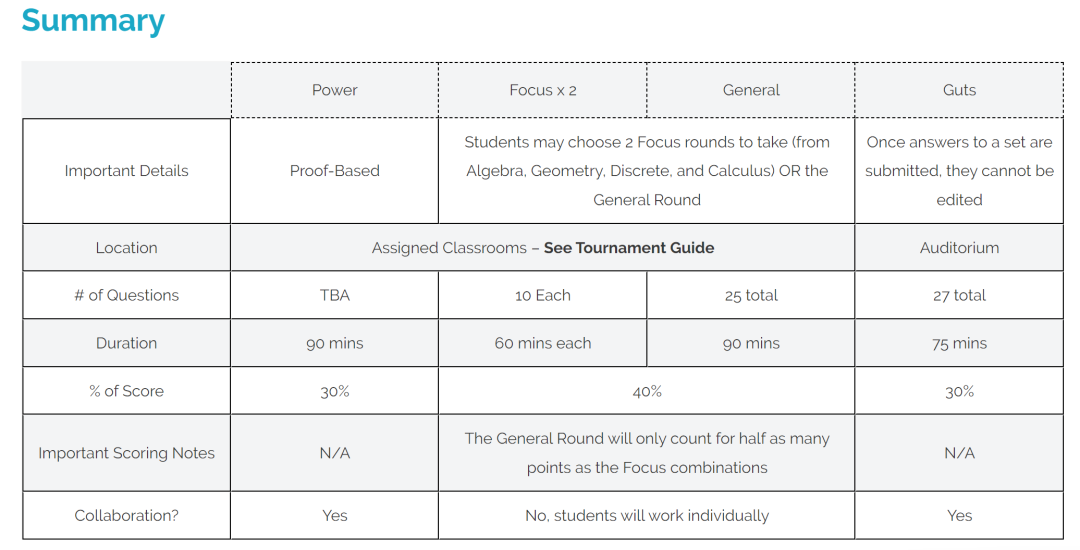# BMT伯克利数学竞赛2023新赛季报名组队中！

①12年级或以下的高中生

②鼓励有能力的低年级学生挑战

1、个人综合挑战赛

*团队每名成员二选一，取团队所有成员的平均分，占团队总成绩的60%

2、个人特长挑战赛

*团队每名成员二选一，取团队所有成员的平均分，占团队总成绩的60%

3、团队力量挑战赛

4、团队车轮战# 翰林（BMT）基础班

1、适合人群：2021年3月目标为BmMT的学生

2、培训班为3-8人小班，满3人开班

3、所有报名课程学员赠送翰林独家精编AMC精编习题集

### 课程大纲

Chapter 01 Perimeter and areas

Chapter 02 Patterns Recognition

Chapter 03 Logical reasoning

Chapter 04 Operations with fractions

Chapter 05 Even and odd

Chapter 06 Word problems related to percent

Chapter 07 Transformations

Chapter 08 Consecutive integers

Chapter 09 Operations with decimals

Chapter 10 Sets and Venn diagram

Chapter 11 Counting techniques

Chapter 12 Divisibility

Chapter 13 Geometric visualization

Chapter 14 Factors

Chapter 15 Prime numbers

Chapter 16 Ratio, rate and proportion

Chapter 17 Least common multiple and greatest common factor

Chapter 18 Solving equations

Chapter 19 Special symbols and operations

Chapter 20 Remainder

Chapter 21 Sequences and series

Chapter 22 Functions

Chapter 23 Pythagorean Theorem and triangles

Chapter 24 Probability

Chapter 25 Angles and triangles

Chapter 26 Rectangles and squares

Chapter 27 Similar triangles

Chapter 28 Trapezoids

Chapter 29 Circles

Chapter 30 Volumes